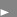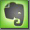Voltampere (VA) or Watts (W)

The power in Watts equals the quantity resulting from the vector product of two vector quantities of voltage and current. If voltage and current are in phase, the resulting power in watts equals the 'in phase'-multiplication of RMS voltage and RMS current. If voltage and current are not in phase because of capacity or inductivity, the resulting power in watts is smaller (the multiplication is not maximum times maximum anymore, for instance).

VA on the other hand is the quantity resulting from multiplying the rms values in volts and amps (VA is a simple abbreviation for Volts time Amperes). This is the voltage and the current that must be provided. The resulting quantity VA can never be smaller than the power that is used by the device under observation.
Watts determine the power that is available for work and VA determines the amount of power needed to supply this 'usable' power. The more reactive the load becomes, the greater is the number of VA required to deliver the same number of watts to the load.
Capacitor Input supplies for instance have the characteristic that the Watt rating is in the range of 0,55 to 0,75 times the Voltampere rating.
See it this way: The VA is what you put in, the Watts are what you get out ...
The ratio of Watt to Voltampere rating is called the Power Factor and is expressed as a number or a percentage (i.e. 0,8 or 80%).
Power Factor = consumed power in Watts divided by the VA necessary to deliver that power. Proper device design should provide a power factor close to 1.0. Devices with significantly low power factors are fan motors and capacitor input supplies.

In a single phase power system: W = VA x pf (power factor).
In a three phase power system loads: W = 1.73 x E x I x pf.

The power factor will only equal 1.0 when the load is purely resistive. Normally the power factor is always a number less than 1.0 and the power in VA is always larger that the power in Watts.

The Power Factor is another term for the cosinus phi.
Power Factor = cos phi

With a fix remaining rms mains voltage, a decreased power factor necessitates a larger rms current to be available to supply the same nominal number of watts to the load and the power supply system must be capable of generating and distributing this disproportionately higher level of current.See: Watts and Volt-Amps: Powerful Confusion, by APC